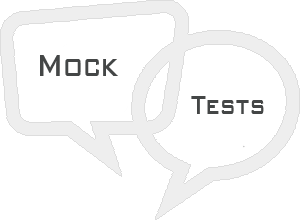# Go Mock Test

This section presents you various set of Mock Tests related to Go. You can download these sample mock tests at your local machine and solve offline at your convenience. Every mock test is supplied with a mock test key to let you verify the final score and grade yourself.# Go Mock Test I

### Explanation

All of the above options are correct.

### Explanation

Both of the above options are correct.

Q 3 - Does Go support type inheritance?

A - yes

B - no

### Explanation

No support for type inheritance.

A - yes

B - no

A - yes

B - no

### Explanation

Q 6 - Does Go support pointer arithmetics?

A - yes

B - no

### Explanation

No support for pointer arithmetic.

Q 7 - Does Go support generic programming?

A - yes

B - no

### Explanation

No support for generic programming.

Q 8 - Is Go a case sensitive language?

A - yes

B - no

### Explanation

Yes! Go is a case sensitive programming language.

### Explanation

Both of the above options are correct.

### Explanation

All of the above options are correct.

### Explanation

Both of the above options are correct.

Q 13 - Can you declared multiple types of variables in single declaration in Go?

A - true

B - false

### Explanation

Yes! Variables of different types can be declared in one go using type inference.

Q 14 - Which of the following is not a boolean type in Go?

A - true

B - false

C - 0

D - All of the above.

### Explanation

0 is not a boolean type.

Q 15 - Which of the following is not a integer type in Go?

A - uint8

B - uint64

C - complex128

D - All of the above.

### Explanation

complex128 is not a integer type.

Q 16 - Which of the following is not a floating type in Go?

A - float128

B - complex64

C - complex128

D - All of the above.

### Explanation

float128 is not a floating type.

Q 17 - Which of the following is a derived type in Go?

A - Pointer types

B - Array types

C - Structure types

D - All of the above.

### Explanation

All of the above are derived types.

Q 18 - Which of the following is a derived type in Go?

A - Union types

B - Function types

C - Slice types

D - All of the above.

### Explanation

All of the above are derived types.

Q 19 - Which of the following is a derived type in Go?

A - Interface types

B - Map types

C - Channel types

D - All of the above.

### Explanation

All of the above are derived types.

### Explanation

All of the above are derived types.

### Explanation

All of the above options are correct.

Q 22 - Which of the following operator gives remainder of after an integer division in Go?

A - ^

B - %

C - \$

D - None of the above.

### Explanation

% − Modulus Operator returns remainder of after an integer division.

Q 23 - Which of the following operator increases integer value by one in Go?

A - ++

B - %

C - --

D - None of the above.

### Explanation

++ − Increments operator increases integer value by one.

Q 24 - Which of the following operator decreases integer value by one in Go?

A - ++

B - %

C - --

D - None of the above.

### Explanation

-- − Decrements operator decreases integer value by one.

Q 25 - Which of the following operator checks if the values of two operands are equal or not in Go?

A - !=

B - <>

C - eq

D - None of the above.

### Explanation

!= − Checks if the values of two operands are equal or not, if values are not equal then condition becomes true.

1 D
2 C
3 B
4 B
5 B
6 B
7 B
8 A
9 C
10 C
11 D
12 C
13 A
14 C
15 C
16 A
17 D
18 D
19 D
20 D
21 D
22 B
23 A
24 C
25 A